SBVS254D February   2018  – August 2019

PRODUCTION DATA.

1. Features
2. Applications
3. Description
1.     Device Images
4. Revision History
5. Pin Configuration and Functions
6. Specifications
7. Detailed Description
1. 7.1 Overview
2. 7.2 Functional Block Diagram
3. 7.3 Feature Description
4. 7.4 Device Functional Modes
8. Application and Implementation
1. 8.1 Application Information
2. 8.2 Typical Application
9. Power Supply Recommendations
10. 10Layout
11. 11Device and Documentation Support
12. 12Mechanical, Packaging, and Orderable Information

• DBV|5
• DQN|4
• YKA|4
• DBZ|3
• DBV|5
• DQN|4

### 6.7 Typical Characteristics

at operating temperature TJ = 25°C, VIN = VOUT(NOM) + 0.5 V or 2.0 V (whichever is greater), IOUT = 1 mA, VEN = VIN, CIN = 1 µF, and COUT = 1 µF (unless otherwise noted)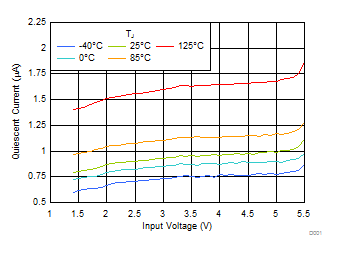VOUT = 3.3 V, includes IQ in dropout
Figure 1. IQ vs VIN and Temperature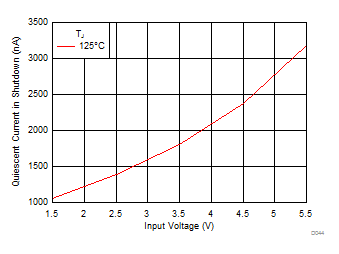VEN < 0.4 V
Figure 3. ISHDN vs VIN and Temperature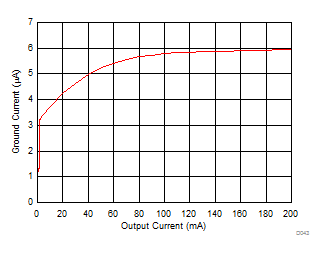Figure 5. IGND vs IOUT up to 200 mA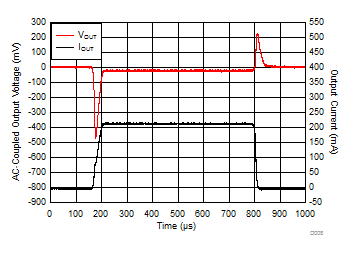Output current slew rate = 6.6 mA/µs
Figure 7. IOUT Transient 0 mA to 200 mA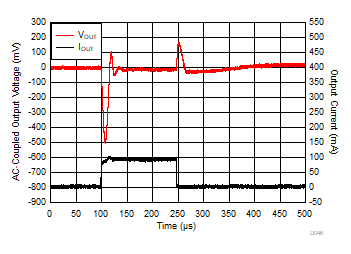Output current slew rate = 100 mA/µs
Figure 9. IOUT Transient 0 mA to 100 mA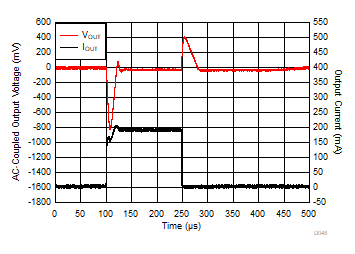Output current slew rate = 200 mA/µs
Figure 11. IOUT Transient 0 mA to 200 mA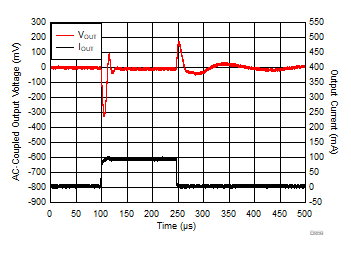Output current slew rate = 100 mA/µs
Figure 13. IOUT Transient 1 mA to 100 mA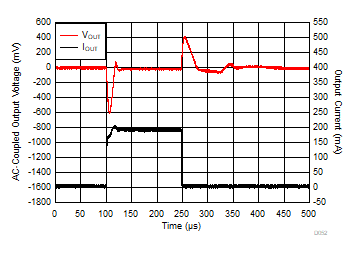Output current slew rate = 200 mA/µs
Figure 15. IOUT Transient 1 mA to 200 mA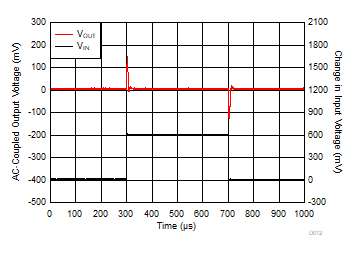IOUT = 100 mA, input voltage slew rate = 0.6 V/µs
Figure 17. VIN Transient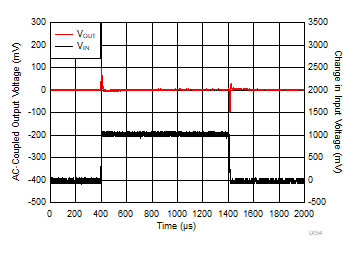IOUT = 150 mA, input voltage slew rate = 0.1 V/µs
Figure 19. VIN Transient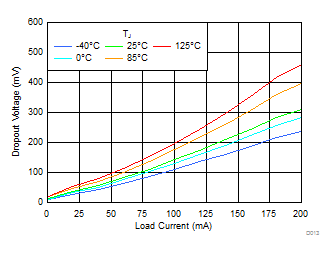VOUT = 1.8 V
Figure 21. Dropout vs IOUT and Temperature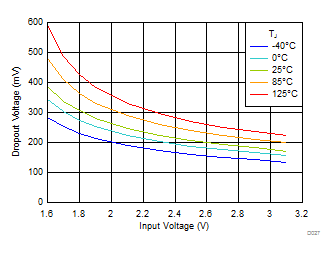Figure 23. Dropout vs VIN and Temperature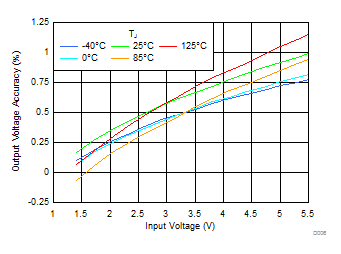VOUT = 0.8 V
Figure 25. Output Accuracy VIN and Temperature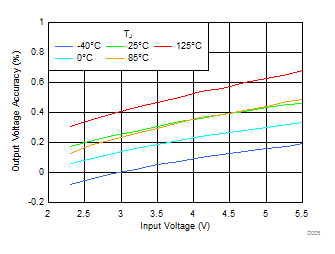VOUT = 1.8 V
Figure 27. Output Accuracy VIN and Temperature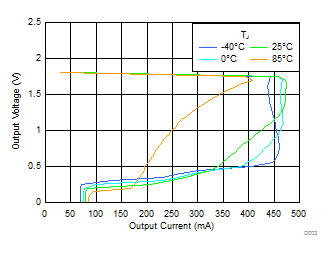VOUT = 1.8 V
Figure 29. Foldback Current Limit vs IOUT and Temperature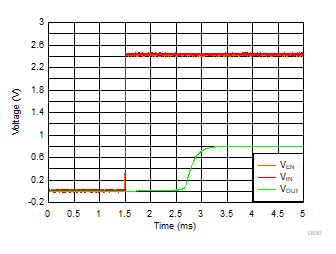VOUT = 0.8 V, IOUT = 30 mA
Figure 31. Startup With VEN = VIN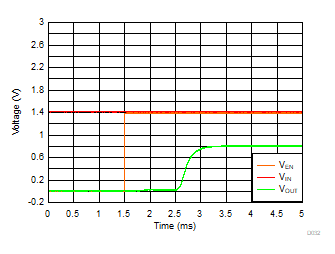VOUT = 0.8 V, IOUT = 1 mA
Figure 33. Startup With Separate VIN and VEN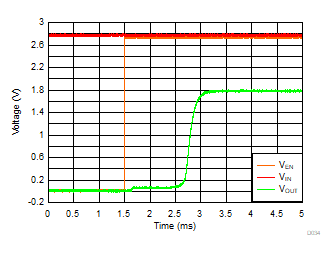VOUT = 1.8 V, IOUT = 30 mA
Figure 35. Startup With Separate VIN and VENVOUT = 1.8 V, IOUT = 200 mA, COUT = 1 µF, CIN = 0 µF
Figure 37. PSRR vs Frequency and VIN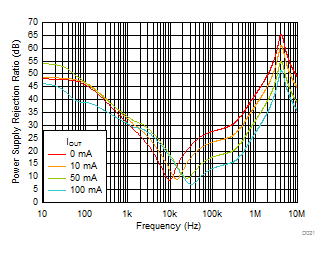VOUT = 0.8 V, VIN = 1.4 V, COUT = 1 µF, CIN = 0 µF
Figure 39. PSRR vs Frequency and IOUT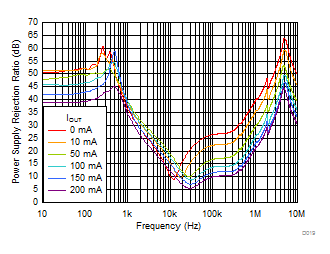VOUT = 3.3 V, VIN = 3.8 V, COUT = 1 µF, CIN = 0 µF
Figure 41. PSRR vs Frequency and IOUTVOUT = 1.8 V, VIN = 2.8 V, COUT = 1 µF
Figure 43. Output Noise vs Frequency and IOUT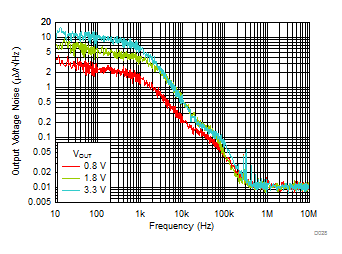VIN = VOUT + 1 V, IOUT = 200 mA, COUT = 1 µF
Figure 45. Output Noise vs Frequency and VOUT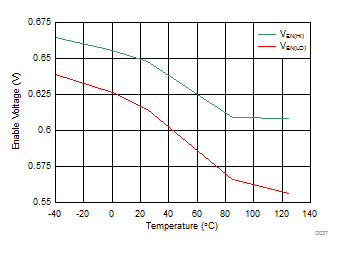Figure 47. Enable High and Low Thresholds vs
Temperature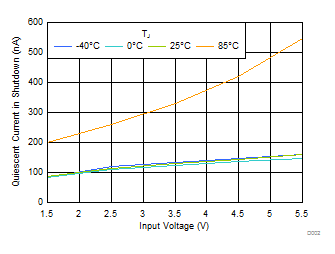VEN < 0.4 V
Figure 2. ISHDN vs VIN and Temperature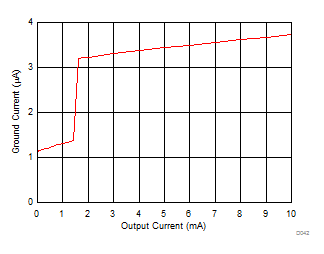Figure 4. IGND vs IOUT up to 10 mA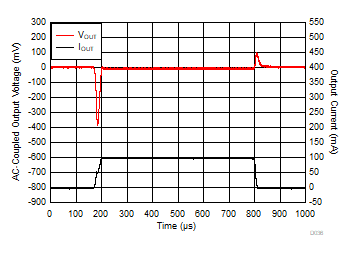Output current slew rate = 3.3 mA/µs
Figure 6. IOUT Transient 0 mA to 100 mA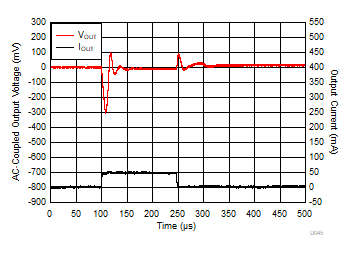Output current slew rate = 50 mA/µs
Figure 8. IOUT Transient 0 mA to 50 mA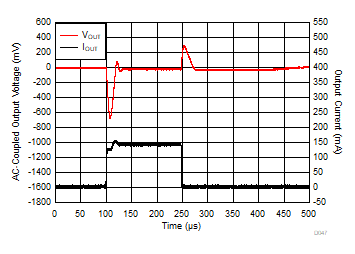Output current slew rate = 150 mA/µs
Figure 10. IOUT Transient 0 mA to 150 mA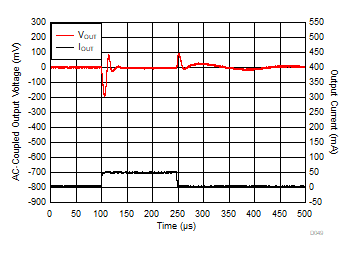Output current slew rate = 50 mA/µs
Figure 12. IOUT Transient 1 mA to 50 mA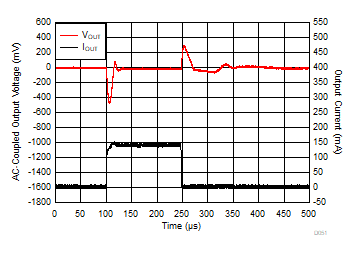Output current slew rate = 150 mA/µs
Figure 14. IOUT Transient 1 mA to 150 mA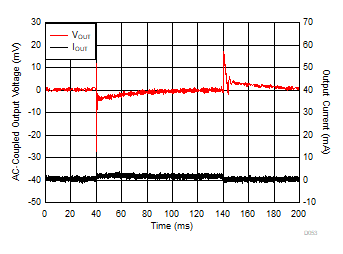CIN = COUT = 10 µF, output current slew rate = 3 mA/µs
Figure 16. IOUT Transient 3 µA to 3 mA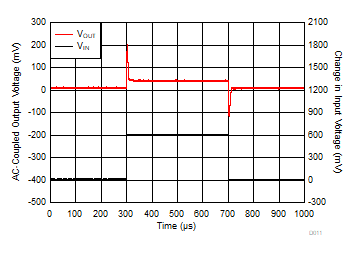IOUT = 200 mA, input voltage slew rate = 0.6 V/µs
Figure 18. VIN TransientIOUT = 20 mA, input voltage slew rate = 0.01 V/µs
Figure 20. VIN Transient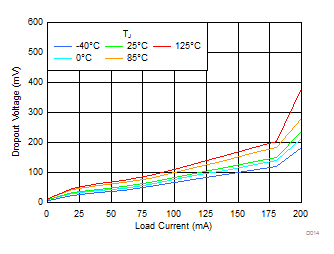VOUT = 3.3 V
Figure 22. Dropout vs IOUT and Temperature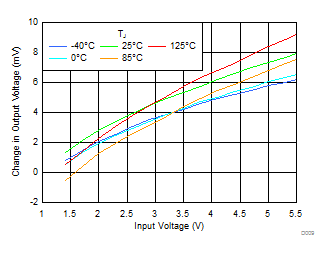VOUT = 0.8 V
Figure 24. Line Regulation VIN and Temperature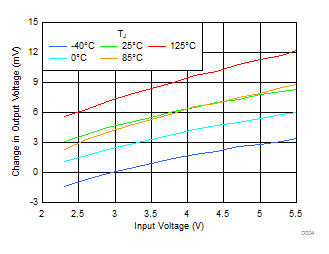VOUT = 1.8 V
Figure 26. Line Regulation VIN and Temperature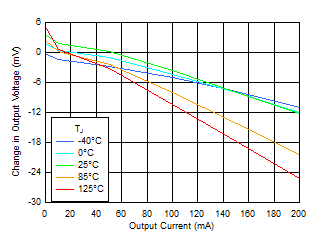VOUT = 1.8 V
Figure 28. Load Regulation vs IOUT and Temperature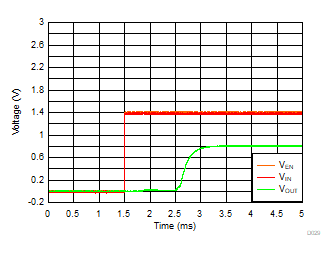VOUT = 0.8 V, IOUT = 1 mA
Figure 30. Startup With VEN = VIN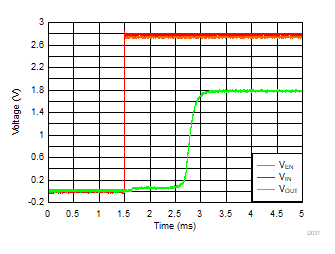VOUT = 1.8 V, IOUT = 30 mA
Figure 32. Startup With VEN = VIN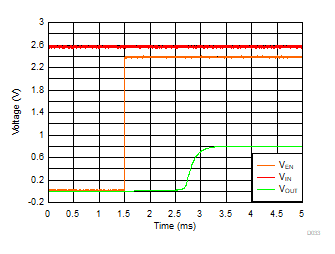VOUT = 0.8 V, IOUT = 30 mA
Figure 34. Startup With Separate VIN and VEN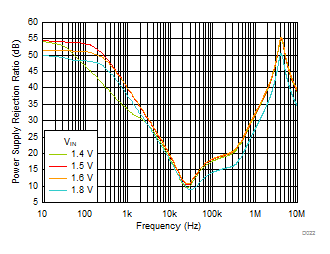VOUT = 0.8 V, IOUT = 200 mA, COUT = 1 µF, CIN = 0 µF
Figure 36. PSRR vs Frequency and VIN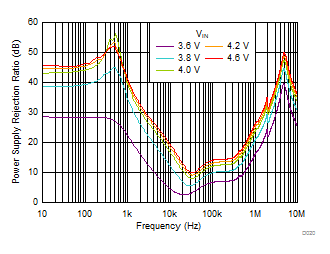VOUT = 3.3 V, IOUT = 200 mA, COUT = 1 µF, CIN = 0 µF
Figure 38. PSRR vs Frequency and VIN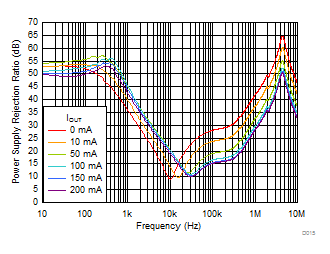VOUT = 1.8 V, VIN = 2.8 V, COUT = 1 µF, CIN = 0 µF
Figure 40. PSRR vs Frequency and IOUT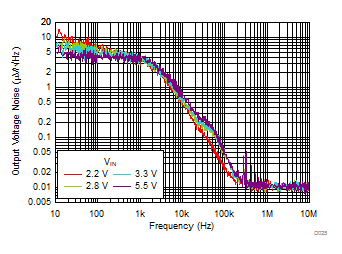VOUT = 1.8 V, IOUT = 200 mA, COUT = 1 µF
Figure 42. Output Noise vs Frequency and VIN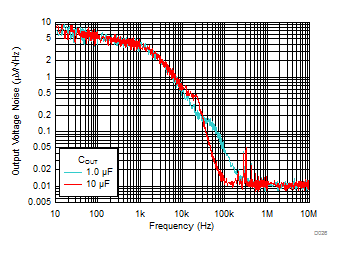VOUT = 1.8 V, VIN = 2.8 V, IOUT = 200 mA
Figure 44. Output Noise vs Frequency and COUT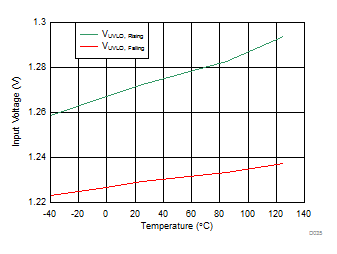Figure 46. UVLO VIN Rising and Falling Thresholds vs Temperature